##### Common Core Math For Parents For Dummies with Videos Online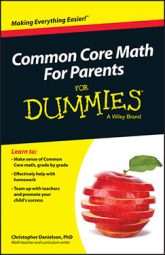While we often think of fractions as "parts of numbers" they're also numbers themselves. Because fractions are numbers, you can compare them. Comparing fractions is an important skill in fourth grade Common Core math.

Some numbers are larger than others; children learn this early on. So asking which of two fractions is larger and putting two sets of fractions in order are sensible tasks.

## Compare denominators

The standard way to compare two fractions is to find equivalent fractions that have the same denominator. To compare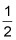and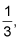you would rewrite them both as sixths: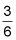and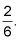Because these two fractions are built of sixths, the fraction with the larger numerator is the larger fraction, so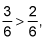and therefore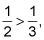as this figure demonstrates.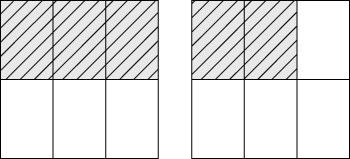Comparing fractions using common denominators

## Compare numerators

Your child may think about this comparison differently, so the following is one more way children often compare fractions — a way that likely comes up in a Common Core classroom.

You can compare fractions using common numerators. In this way of thinking, you don't need to change the forms ofand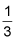in order to compare them. With each of these fractions, you have one piece. The question to think about is "How big are the pieces?" If you partition something into two equal pieces, you have bigger pieces than if you partition the same thing into three equal pieces. Partitioning into two equal pieces means each piece isof the original whole. Partitioning into three equal pieces means each piece isof the original whole. Therefore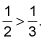A more complicated example could be comparingand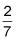using common numerators. You can rewriteas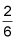so that it has a 2 in the numerator just likedoes. Sixths are bigger than sevenths, so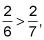and so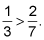The next figure shows this problem on the number lines.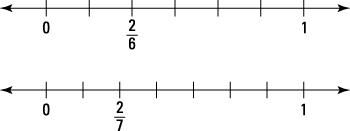Comparing fractions on number lines.